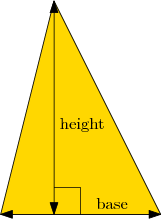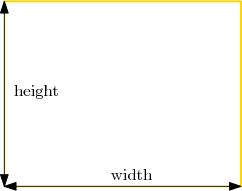# 10: def

In this lesson we show you the most important idea in programming: defining your own functions! Functions let your program become shorter, better-organized, easier to read, easier to debug, and more reusable. In later lessons we will see other benefits like recursion.

# Defining a Function

We'll start with an example. Remember the function `abs`? It takes one argument (a number x), and returns its absolute value (which is x when x ≥ 0, and -x when x < 0). The way to define this function in Python is:

```def abs(x):     # define a function named 'abs' of one argument named 'x'
if x >= 0:    # function body starts here
return x
else:
return -x   # function body ends here```

## The Two Parts of a Function Definition

The first line in a function definition specifies the name of the function and the arguments of the function. It should look like

`def «function-name»(«argument-list»):`

In the example above, `abs` was the function name, and the argument list was `x`. It is called an argument list because there can be multiple arguments such as `x, y, z`; it's also possible to have zero arguments, i.e. an empty list. The arguments are the input(s) to the function.

Everything after the first line of a function definition is the body of the function. The body is an indented block of code. Whenever the function is called, the body is executed on those arguments/inputs. Finally, when we reach this line in the function body,

`return «value»`

the function stops running and returns `«value»` as its output. That is, the return value is the output of the function. As the `abs` example shows, the body may contain several `return` statements; but only the first one executed has an effect since the function stops executing afterwards.

## Example: Defining and Calling a Function

The following program contains a function definition and some other statements that are executed after the function is defined. Can you guess what the output will be?

We see that the function name is `square`, there is just one argument `x`, and that the function body consists of just one line `return x*x`. Then outside of the function we have two commands, which call the function a total of three times.

As the visualizer shows, note that a separate chunk of working space (a frame) is allocated each time the function is called. Once the function returns, that working space is no longer needed, and erased.

Here are the steps explained in words:

1. When the first command is executed, Python must evaluate `square(10)`.
• Python compares the list of inputs `(10)` to the argument list `(x)`. So, it executes the function body `return x*x` while remembering that `x` equals `10`. Thus `x*x` yields `100`, which is printed.
2. When the second command is executed, Python must evaluate `square(square(2))`.
• The inner part `square(2)` is evaluated first. We temporarily set `x` equal to `2` and run the function body, which returns `4`.
• Then the outer expression is evaluated; since `square(2)` gave `4`, we are now calling `square(4)`. This returns `16`, which is printed.

## Four Common Mistakes

One common mistake you can make in defining a function is to forget the `return` statement.

Example
Mistake 1: forgetting to `return`

As you can see, if no `return` statement is encountered in the body, then the function gives `None` by default. So, if you are failing some exercise because a function is returning `None`, the problem is often that some function inputs are not causing a `return` statement to be executed.You may also intentionally omit a `return` statement. This only makes sense if your function has some side effect other than its return value, for example printing some output:
Example: A side effect and no return statement

Another common mistake is forgetting to indent the code, resulting in an `IndentationError`.

Example
Mistake 2: forgetting to indent

As we saw in lesson 2, calling with too few or too many arguments causes an error.

Example
Mistake 3: call with too many arguments

Finally, if you make a typo when defining or calling the function, you will get an error saying that the function is undefined.

Example
Mistake 4: wrong name

## Try it YourselfThere's no need to use `input()` or `print()` for this exercise, or for future exercises where you're asked to "define a function." The grader will call your function with arguments automatically, and inspect the result it returns directly. A correct solution for this particular exercise will be 2 lines (see the 'The Two Parts of a Function Definition' at the top of the lesson).Coding Exercise: Cubism
Define a function `cube(n)`, which takes a single number `n` as input, and outputs its cube `n` × `n` × `n`.
Enter testing statements like `print(myfunction("test argument"))` below.

## Two or More ArgumentsThe functions above only took one argument, but a function can be designed to take any number of arguments. For example, you have been calling `input()` with 0 arguments (and you'll define a function with zero arguments in lesson 15A).

Here's an example with two arguments, about geometry. Suppose we need a function to compute the area of a triangle, given the length of its base and its height. The formula for the area is

area = base × height / 2

In code, this looks like the following: we replace `def «function-name»(«argument1»)` with `def «function-name»(«argument1», «argument2»)`.

Another important thing to note is that when a function is defined is different from when the function is executed. The body doesn't run until the function is actually called. To test this, complete the following exercise:Multiple Choice Exercise: In-n-Out
What is the output of the following program?

`def f():          # function of 0 arguments   print("in f")print("not in f")f()               # call f now`
Correct! The function `f` is defined first of all, but its body is not executed immediately. After the function is defined we print out `not in f`. Then the function is called, and the body is executed, printing `in f`.In the last exercise we ask you to write your own function of two arguments, to compute the perimeter of a rectangle. (The perimeter is the total length of all sides.) In a rectangle with a given width and height, its perimeter is given by the following formula:$\textrm{perimeter = width + height + width + height}$

See the diagram at right for an example.Coding Exercise: Rectangle
Define a function `rectanglePerimeter(width, height)` that returns the perimeter of a rectangle.
Enter testing statements like `print(myfunction("test argument"))` below.

### Functions Calling Functions

Functions are the building blocks of well-built large programs: you can do the same task twice without writing out the same code twice, and you can re-use solutions to common tasks. Here is an example of building one function and using it inside of another one.Can you guess what this program does, before you run it? Remember from lesson 4 that multiplication of strings and integers means to repeat the string that many times. For example, `"tar"*2` is `"tartar"`.

Drag the vertical gray line left and right to change how much code & visualization is shown.

You can see a new phenomenon in the visualization: under the "Frames" column, when we are at Step 8 of 30 in the execution, there are not only some global variables, but two frames (one for `outer`, and one for `inner` that was just created). The `outer` one stays waiting until the `inner` one is completely finished, then the `outer` one resumes. It happens to make another call to `inner`, and again `outer` waits; and when `inner` is finished, then `outer` continues to completion. Finally, we call `outer` and the whole process is repeated.

You are now ready for the next lesson!